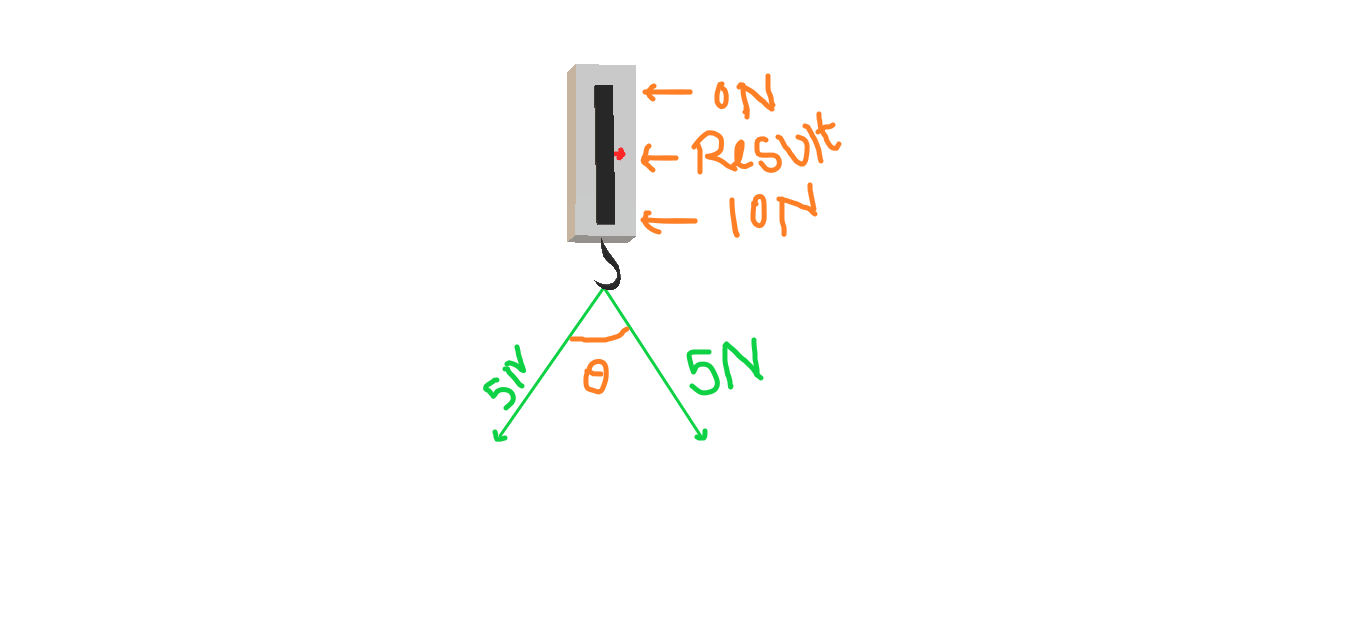#### Vectors

2

Before proceeding to explain vectors, I’ll like to ask a simple question, “Do you know what physical quantity is ?”. If answer is – ‘Yes’, we are safe to go ahead but in other scenario I’ll recommend reading Physical Quantities.

If we closely watch physical quantities, we can categorized them in two. Yes this is true. Let me start with an example. I am picking up two physical quantities- Force and Mass.

Let’s first consider mass. Suppose we have two blocks of masses, 5 kg ( of course this is random example ) each. Now suppose we want to add these two blocks to add to make one. If we join them from any direction, any angle the joint magnitude of mass is 10 kg, without being affected by the direction or angle from which two were added.

So we can make a conclusion that addition of mass does not depend on direction.

## Is this conclusion true for every physical quantity?

Keeping in mind the above question, think about Force. Suppose a situation where two forces are simultaneously applied on a body, each having a magnitude of 5 N. What about the resulting force? Will it always be 10 N?

You can get answer to these questions by performing a very simple experiment. The requirement for the experiment is only a spring balance.As shown in the figure, apply two forces of ( I assume you can make the set up for applying forces ) 5 N each. Now vary the angle between two forces and monitor the readings in spring balance. And here is the boom! If you don’t know this already you are going to be surprised. You will notice that addition of two force may result in anything between 0 to 10 N ( in this case, of course ). For a generalized result we can conclude-

#### Addition of two forces may result in any thing between 0 to sum of their magnitudes depending on direction.

So when we add two forces of 5 N each, the result may be 10 N or 0 N or anything between 0 N to 10 N depending on the direction between two forces. And of course this is not the case of algebra. We all know ( at least you are reading this article and reached this point, I can assume you know basic algebra ) that algebra doesn’t allows 5+5=0 or anything like 5+5 ≠ 10. What I want to point out is that here the addition of forces doesn’t follow the law of algebra.

That means force is not identical with mass. Depending on this basis, we can categorize whole physical quantities in two categories. One who fall in the group of mass are called scalars. And others who fall in the group of force are called vectors.

2

•#### manish ojha

that’s interesting sir but I’m familiar with it previously as u taught me this earlier in same manner & Sir I wanna ask u 1 qs related to blog writing as I also want to write blog but couldn’t get info. so pls.. sir again guide ur Obedient Student non other than Manish Ojha
Thanking u 🙏

•#### Ravi Kant Singh

Sure Manish, I’m always here to help my students. You can ask anything, I’ll sure answer if I have.

•#### manish Ojha

I’m familiar with this fact Sir as u earlier taught me same bt it’s interesting as per ur view i.e “if I read something again & again it some new inventory knowledgable thing arise

•#### fayrowe

Hi there, I found your site via Google while looking for a comparable topic,
your site got here up, it seems good. I’ve bookmarked it in my google bookmarks.

Hi there, simply changed into aware of your blog thru Google, and found that
it’s truly informative. I am gonna be careful for brussels.
I will appreciate should you continue this in future. A lot of other people
will probably be benefited out of your writing.
Cheers!